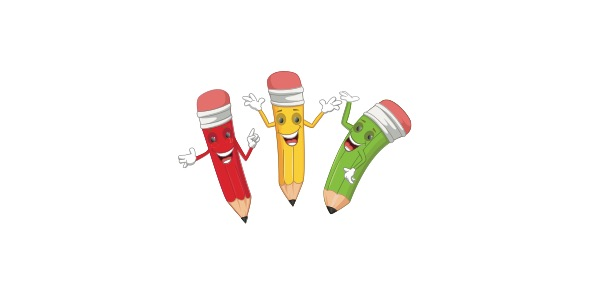# Quiz On 3rd Grade Rounding

10 Questions | Total Attempts: 585SettingsTake this cool and interesting quiz to see how well you know the number rounding. Let's find out now by taking this quiz.

Related Topics
• 1.
Round 253 to the nearest tens.
• A.

240

• B.

300

• C.

250

• D.

200

• 2.
Round 817 to the nearest hundreds.
• A.

810

• B.

800

• C.

820

• D.

900

• 3.
What digit do you need to LOOK AT to round 462 to the nearest tens?
• A.

4

• B.

6

• C.

2

• 4.
What is 296 rounded to the nearest hundreds?
• A.

300

• B.

200

• C.

290

• D.

260

• 5.
What is 781 rounded to the nearest tens?
• A.

700

• B.

710

• C.

800

• D.

780

• 6.
Round 3,278 to the nearest thousand.
• A.

3,000

• B.

4,000

• C.

3,300

• D.

3,280

• 7.
Round 8,264 to the nearest hundred.
• A.

8,000

• B.

8,200

• C.

8,260

• D.

8,300

• 8.
Take the number 2,794 and round it to the nearest ten.
• A.

2,800

• B.

2,790

• C.

3,000

• D.

2,700

• 9.
If rounding 1,208 to the nearest hundred, what place value would you look at to make your decision?
• A.

Ones

• B.

Thousands

• C.

Tens

• D.

Hundreds

• 10.
Round the number 5,631 to the nearest hundred.
• A.

5,000

• B.

5,700

• C.

5,630

• D.

5,600